Want to share your content on R-bloggers? click here if you have a blog, or here if you don't.

On the previous post we showed why modules are usefull to build Shiny applications. We also saw a first minimal “Hello-World” example.

It can get difficult to share reactive from/to modules. On this post we will see the 3 most common use cases of data workflow:

• Module → Application
• Application → Module
• Application ↔ Module

## Want to run the examples ?

All code used in this post are available in Github ardata-fr/Shiny-Modules-Tutorials. This repository is actually an R package containing all the modules. Applications are stored in the folder `inst`.

To get apps locally, install the package and run applications:

```# install.packages("remotes")
remotes::install_github("ardata-fr/Shiny-Modules-Tutorials")
library(shinyModulesTuto)
# List available applications
listEx()
# Run first application
runEx(listEx())```

## Data from Module to Application

Online application here or get it locally with:

```# Run Shiny application
runEx("module-vs-app.R")```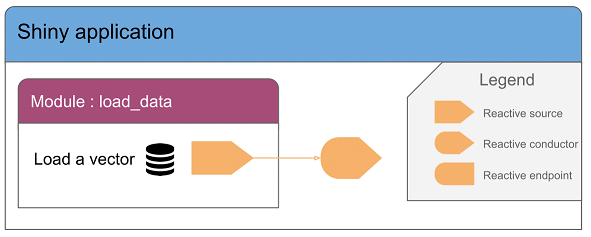### In the module

The `reactiveValues` returned is initialized as below:

```toReturn <- reactiveValues(
variables = NULL,
variable_name = NULL,
trigger = 0
)```

It will be updated as a standard `reactiveValues`:

```observeEvent(input\$AB_load, {
toReturn\$variable       <- get(input\$SI_dataset)[,input\$SI_var]
toReturn\$variable_name  <- input\$SI_var
toReturn\$trigger        <- toReturn\$trigger + 1
})```

Then the result is returned to application with:

`return(toReturn)`

### In the application

Get results from module with:

```# results is created as a reactiveValues with 3 slots (returned from module)
results <- callModule(module = load_data, id = "id1")```

Used in application as a standard `reactiveValues`:

```output\$PR_results_print <- renderPrint({
print(results\$variable_name)
print(results\$variable)
})```

## Data from Application to Module

Online application here or use command:

```# Run Shiny application
runEx("app-vs-module.R")```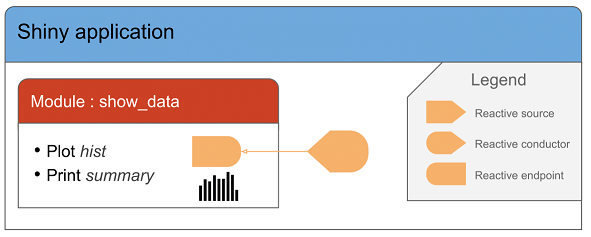### In the application

#### Solution 1: Using a reactive

The `reactive` given to module is created as below:

```variable <- reactive({
iris[, input\$SI_colname]
})```

Pass the `reactive` as a module parameter:

```callModule(module = show_data, id = "id1",
variable = variable,
variable_name = reactive(input\$SI_colname))```

NB : As `variable` is a `reactive`, no need to use the function `reactive()`.

#### Solution 2: Using reactiveValues

The `reactiveValues` given to module is created as below:

```rv <- reactiveValues(variable = NULL)

observe({
rv\$variable <- iris[, input\$SI_colname]
})```

Pass the `reactiveValues` as a module parameter:

```callModule(module = show_data, id = "id2",
variable = reactive(rv\$variable),
variable_name = reactive(input\$SI_colname))```

NB : As `rv\$variable` is a `reactiveValues`, we must use the `reactive()` function.

### In the module

#### Solutions 1 & 2

For both solutions using a `reactive` or a `reactiveValues` in the application, you can get parameters from the module using:

```output\$PL_histogram_var <- renderPlot({
hist(variable(), main = variable_name(), xlab = NULL)
})```

NB : We can use module parameters `variable` & `variable_name` as a standards `reactive` (eg `variable()` & `variable_name()`).

## Data from Application modified in Module

Online application here or use command:

```# Run Shiny application
runEx("app-pong-module.R")```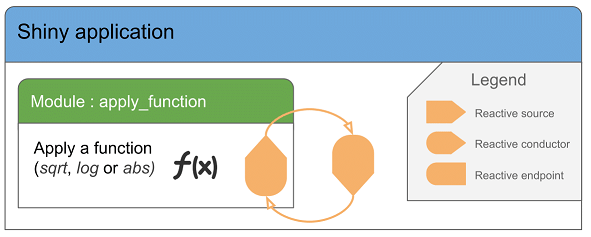How can I get data in my application and let a module modify them ? The trick here is to use a second `reactiveValues`.

We saw on the previous chapter how to deal with parameters inside modules. On this part, we will focus on the application.
Let’s consider the module `apply_function` that takes as parameter a numeric vector on which the user can apply a function (ex : `log(x)`). The module returns a `reactiveValues` with 3 slots : the new numeric vector, the function name used and a “trigger” increasing when the user applies a function.

In the application we initialize the `reactiveValues` as usual:

```rv <- reactiveValues(variable = NULL, fun_historic = NULL)

observe({
rv\$variable     <- iris[, input\$SI_colname]
rv\$fun_historic <- NULL
})```

Then pass it to the module `apply_function`:

```modified_data <- callModule(module = apply_function, id = "id1",
variable = reactive(rv\$variable))```

The output of module `apply_function` is stored as a `reactiveValues`.
It returns contains 3 slots:

• result (the result of the function applied)
• fun (name of the function applied)
• trigger (integer that increases when user applies a function)

In the application, to update our `rv\$variable` according to `modified_data\$result`, we set an `observeEvent` on `modified_data\$trigger`:

```observeEvent(modified_data\$trigger, {
rv\$variable     <- modified_data\$result
rv\$fun_historic <- c(rv\$fun_historic, modified_data\$transformation)
})```

The key here is to use a second `reactiveValues`. So the real schema should be: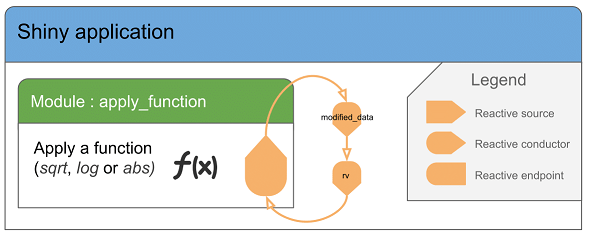## Application combining all previous examples

Online application here or use command:

```# Run Shiny application
runEx("whole-app.R")```

This application combines all the modules created so far. The module `apply_scale` adds a second way of modifying the `rv` “main” reactiveValues with the function `scale`. The schema below shows that the reactiveValues `rv` is the core of the application. It contains 2 slots, `variable` which is the numeric vector and `fun_history` containing the list of functions applied on our numeric vector.

Within the application, the reactiveValues `rv` is created as below:

`rv <- reactiveValues(variable = NULL, fun_history = NULL)`

Then it’s updated by the 3 modules:

• By module load_data using the temporary reactiveValues `data_mod1`.
• By module apply_function using the temporary reactiveValues `data_mod2`.
• By module apply_scale using the temporary reactiveValues `data_mod3`.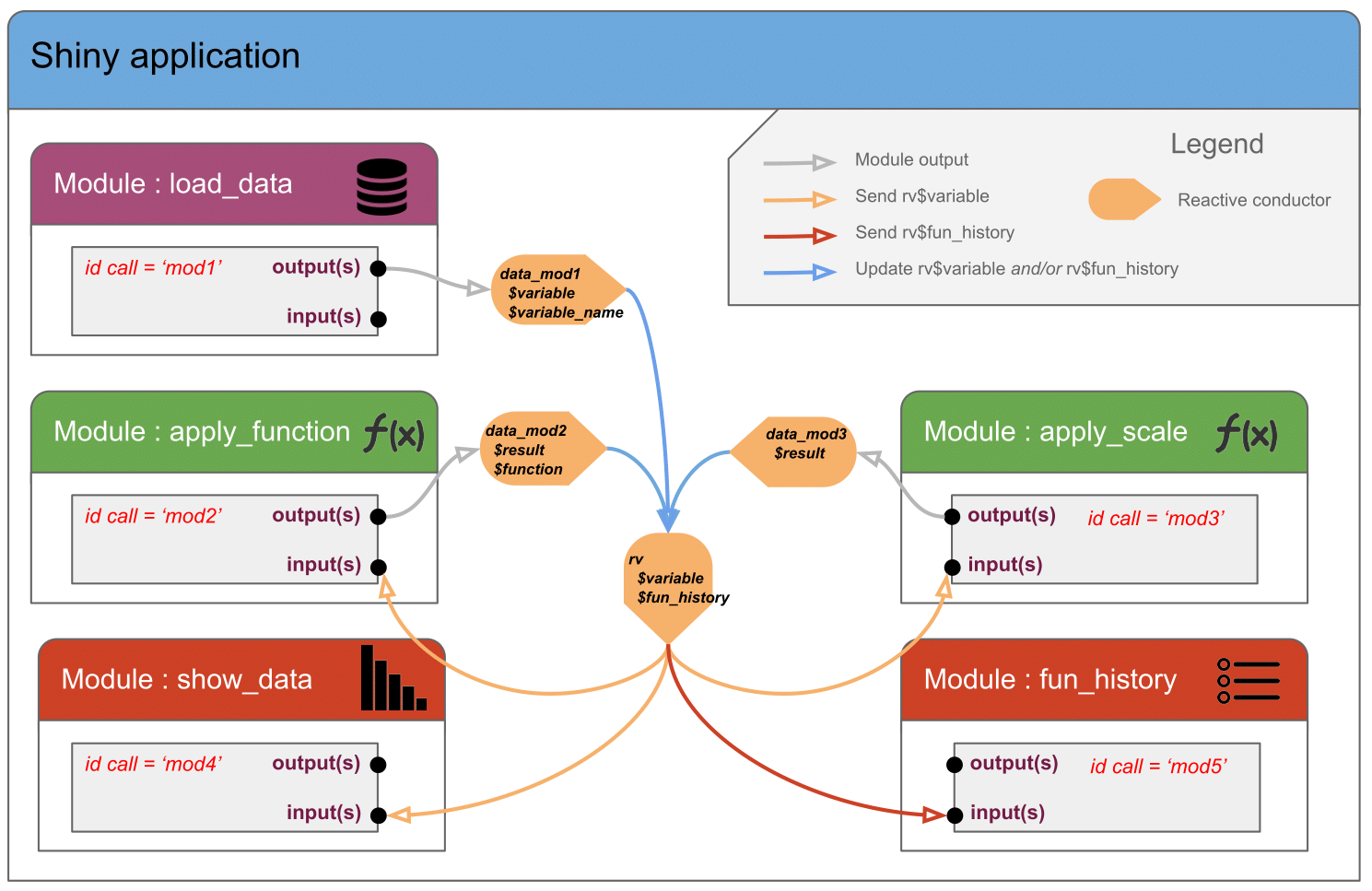To ease the process of initializing/updating the `rv` reactiveValues, `observeEvent` are set on the `trigger` slot returned by modules. Thus we’re sure that the last user action is taken into account.

Trigger the load or reload from module `load_data`:

```# Call the module load_data
data_mod1 <- callModule(module = load_data, id = "mod1")

# When data_mod1\$trigger changes, (re)initialization of rv\$variable & rv\$fun_history
observeEvent(data_mod1\$trigger, {
req(data_mod1\$trigger>0)
rv\$variable    <- data_mod1\$variable
rv\$fun_history <- c()
})```

Trigger the modification through module `apply_function`:

```# Call the module apply_function with parameter rv\$variable
data_mod2 <- callModule(module = apply_function, id = "mod2",
variable = reactive(rv\$variable))

# When data_mod2\$trigger changes, update of rv\$variable & rv\$fun_history
observeEvent(data_mod2\$trigger, {
req(data_mod2\$trigger>0)
rv\$variable    <- data_mod2\$result
rv\$fun_history <- c(rv\$fun_history, data_mod2\$fun)
})```

Trigger the modification through module `apply_scale`:

```# Call the module apply_scale with parameter rv\$variable
data_mod3 <- callModule(module = apply_scale, id = "mod3",
variable = reactive(rv\$variable))

# When data_mod3\$trigger changes, update of rv\$variable & rv\$fun_history
observeEvent(data_mod3\$trigger, {
req(data_mod3\$trigger>0)
rv\$variable    <- data_mod3\$result
rv\$fun_history <- c(rv\$fun_history, "scale")
})```

## Conclusion

We described here (links to applications):

We highly recommand using modules when building complex/large Shiny applications.
But there’s even more if you want to go further:

• Nested modules (which is actually not complicated at all)
• Dynamic module calls

Before seing this concepts (let’s write a part 3 !!) I hope you will have great time developping your Shiny applications !